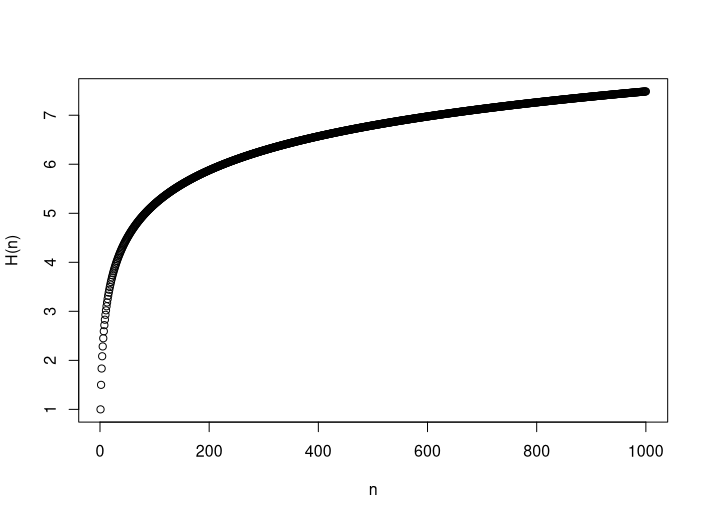# Breaking Pills: An Intuition

Posted on Sep 23, 2019

### Motivation

Recently, Mark Dominus’ post on breaking pills appeared on Hacker News. I was surprised to see the author not provide a closed-form and wanted to extend on some of the comments indicating a relation to the harmonic numbers.

### Problem Formulation

Starting off with a bowl of $$n$$ whole pills, you draw from the bowl uniformly at random according to the following mechanism: if the drawn pill is whole, you put back one half of it; if the drawn pill is half, you don’t put back anything.

How many half pills will be left in the bowl once there are no more whole pills?

### Solution

Let us first state some useful observations:

• Every whole pill will be drawn eventually and sequentially. We can hence assume an order on the whole pills, wlog.
• Every whole pill can be associated with exactly one half pill that will be put back into the bowl. Moreover, every half pill has to be associated with exactly one whole pill, i.e. it is a 1-to-1 mapping.
• Instead of asking the more general question of ‘How many half pills will be left?’ we can ask the more specific question of ‘For how many whole pills, does the associated half pill remain in the bowl?’.
• For such a half pill to remain in the bowl, it has to be picked after (or not at all, depending on how you phrase the problem) all remaining whole pills.

For the sake of concreteness, let us illustrate the mechanism with $$n=7$$. Recalling that we can impose an order on whole pills, we look into the third whole pill W3 and the half pill h3 associated with it. More precisely, we look into h3’s position relative to all remaining whole pills. We can safely forget about the previous whole pills. These are the possible positions:

W3 | h3 | W4 | W5 | W6 | W7 W3 | W4 | h3 | W4 | W6 | W7 W3 | W4 | W5 | h3 | W6 | W7 W3 | W4 | W5 | W6 | h3 | W7 W3 | W4 | W5 | W6 | W7 | h3

Note that only in the last scenario, does the half pill add to the count of half pills remaining after all whole pills. Hence, in expectation, the half pill associated with the first whole pill adds $$\frac{1}{5}$$ to the count of remaining half pills.

This ordering argument can be applied to all subsequent (as well as previous) whole pills: just with fewer (or more) whole pills to position. The last pill, representing the base case, will always add 1 half pill to the counter as there is only one position to choose from.

Applying our observation to all of the $$n$$ whole pills we obtain:

\begin{aligned} N_n &= \text{\#half pills left after the draw of } n \text{ whole pills} \\ \mathbb{E}[N_n] &= \sum_{i \in [n]} \Pr[\text{half pill from } i \text{th whole pill remains until the end}] \\ &= \sum_{i \in [n]} \Pr[i\text{th half pill is positioned after remaining whole pills } i + 1, i+2, \dots n] \\ &= \sum_{i \in [n]} \Pr[i\text{th half pill is positioned at last of } i \text{ positions}] \\ &= \sum_{i \in [n]} \frac{1}{i} \\ &= H_n \end{aligned}

In case such a (hopefully) intuitive reasoning still seems unsatisfactory, note that the given argument can easily be applied in a more explicit proof by induction.

### Closing remarks

Recall that the harmonic number can be very well approximated by the natural logarithm. In particular, we have $$H_n = \Theta(\log(n))$$ and $$\gamma + \log(n) < H_n < \gamma + \log(n+1)$$, where $$\gamma \approx .577$$ is the Euler-Mascheroni constant.Thanks to Philip for bringing this problem to my attention.